# Printable Multiplication Chart 100 XUpdated on Oct 10, 2020By Printablee Team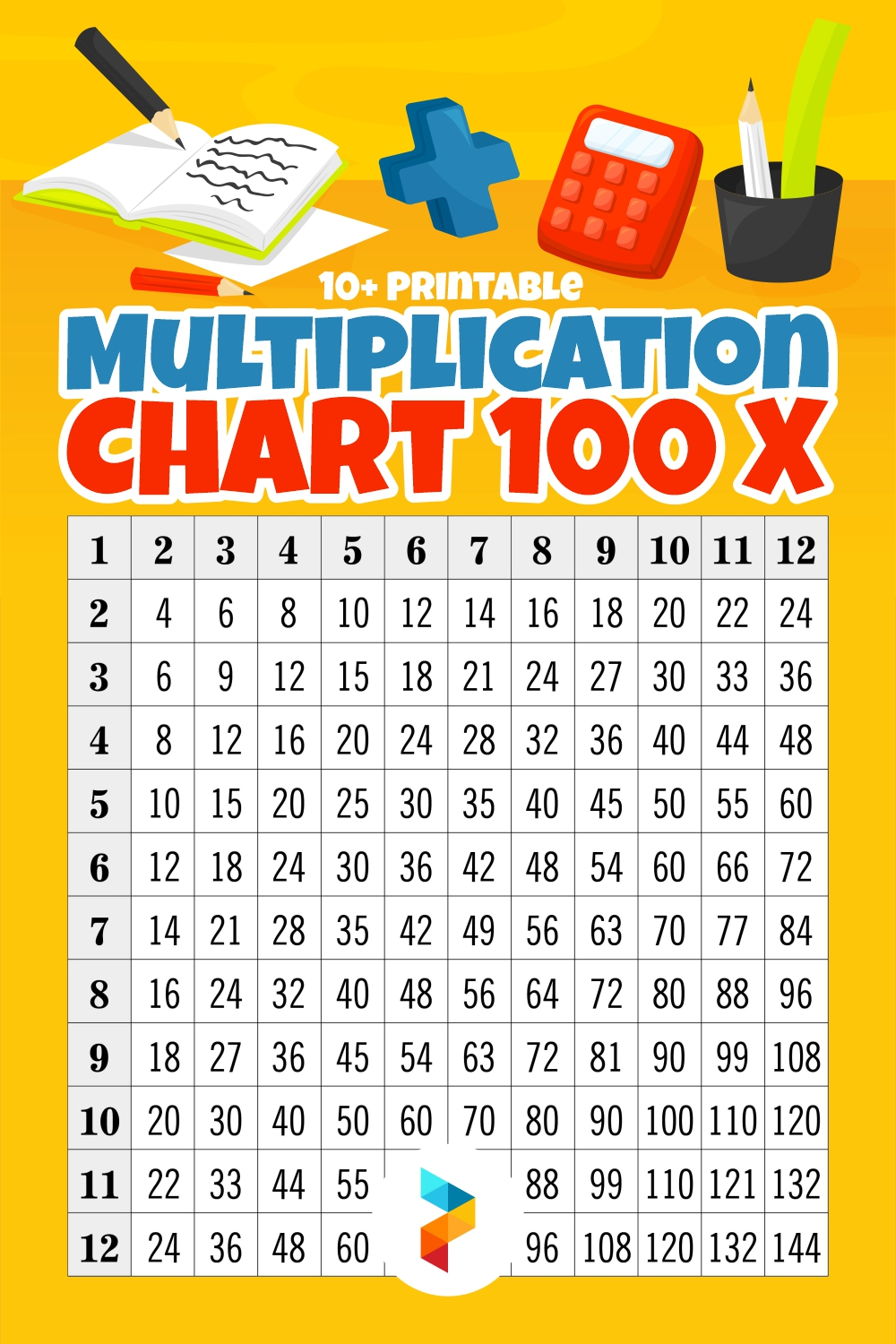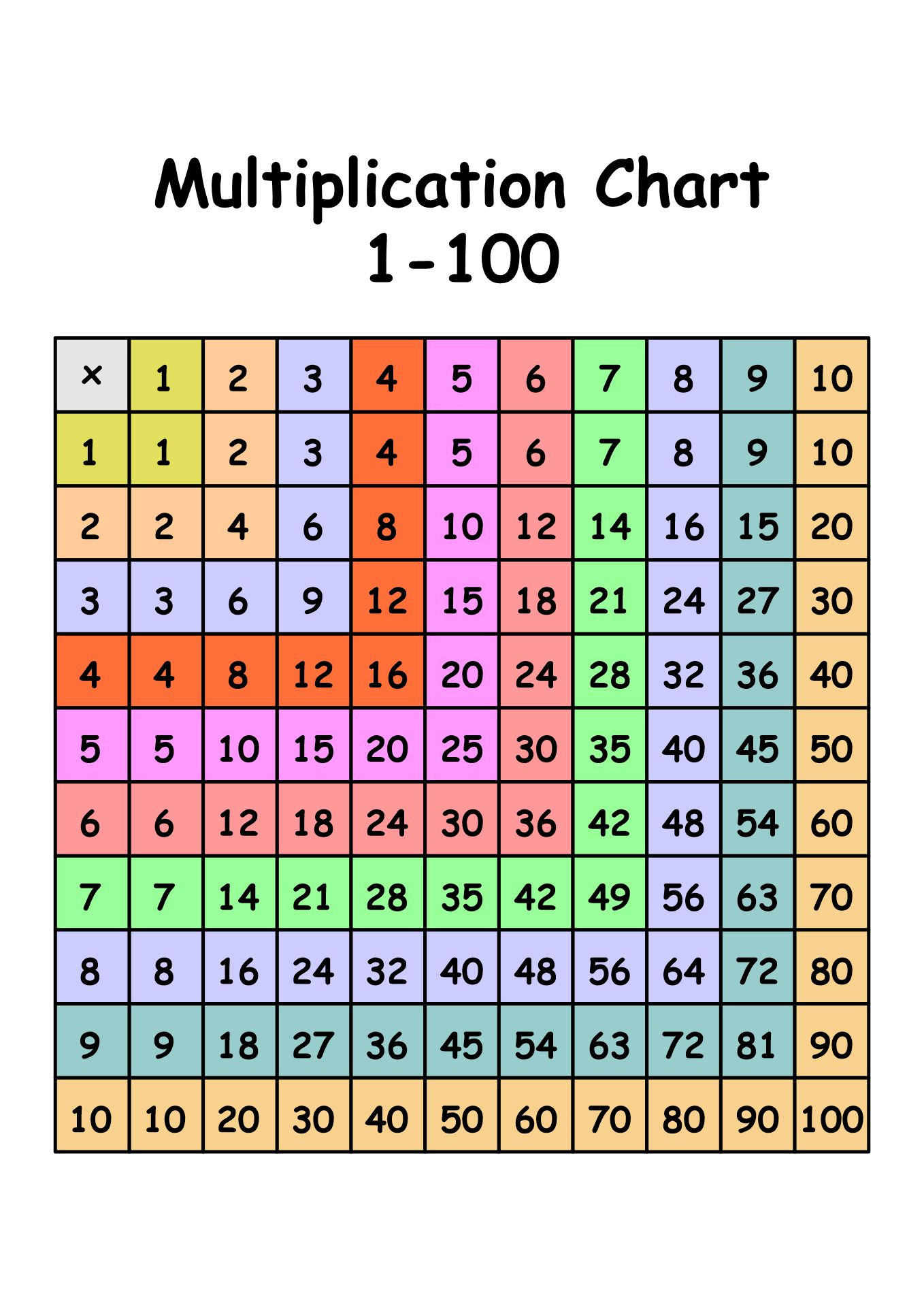## How to Learn Multiplication?

The arithmetic action of multiplication combines two or more integers to produce their result. Understanding multiplication is crucial for many different mathematical and practical computations since it is a fundamental mathematical concept with many applications.

Numerous situations in daily life, mathematics, and other disciplines use multiplication. It is used to figure out ratios and proportions, scale numbers, calculate areas and volumes, and solve mathematical issues.

Additionally, the groundwork for more complex mathematical ideas like algebra, calculus, and higher-level problem-solving is laid through multiplication.

### What is the basis of mathematics?

A basic mathematics process called multiplication entails merging two or more integers to produce their output. It involves adding a number to itself a certain number of times repeatedly.

The numbers being multiplied are referred to as the factors in multiplication, while the outcome is referred to as the product. The "x" symbol or lining up the components without any additional symbols designates the multiplication process.

### What are the common methods for performing multiplication?

The approach selected will rely on the quantity of data being used, the needed level of precision, and personal taste. These techniques can increase the precision and speed of multiplication computations.

• Traditional Algorithm: The traditional algorithm, also referred to as long multiplication, is frequently used to multiply numbers with multiple digits. It entails starting from the rightmost digits and multiplying each digit of one number by each digit of the other number, carrying over any remainder.

• Grid Method: When multiplying numbers with multiple digits, the grid method is useful. It entails making a grid and placing each factor on the top and left of the grid. The products of the corresponding digits are used to fill in the intersections within the grid, and the sum of the products is used to determine the outcome.

• Method of Partial Products: This technique divides multiplication into smaller calculations. Each digit of one number is multiplied by each digit of the other, the partial products are noted, and the final result is then added to the total.

• Mental Math Techniques: Multiplication can be swiftly and mentally accomplished using mental math techniques. These methods make use of number patterns and attributes to make calculations simpler. Mental multiplication can be made simpler by separating numbers, doubling and halving, or employing established multiplication facts.

• Lattice Multiplication: This visual technique entails the construction of a grid or lattice structure. The crossings within the grid are utilized to calculate the partial products. The digits of the factors are positioned along the top and right sides of the grid. The final product is then created by adding diagonally the partial components.

• Estimation: Without performing an exact calculation, estimation is a technique used to acquire a general sense of the outcome. It entails simplifying the numbers by rounding them, mentally multiplying the rounded numbers, and changing the outcome according to the degree of rounding. The estimation might be helpful for quickly determining whether an answer is reasonable or for carrying out quick computations.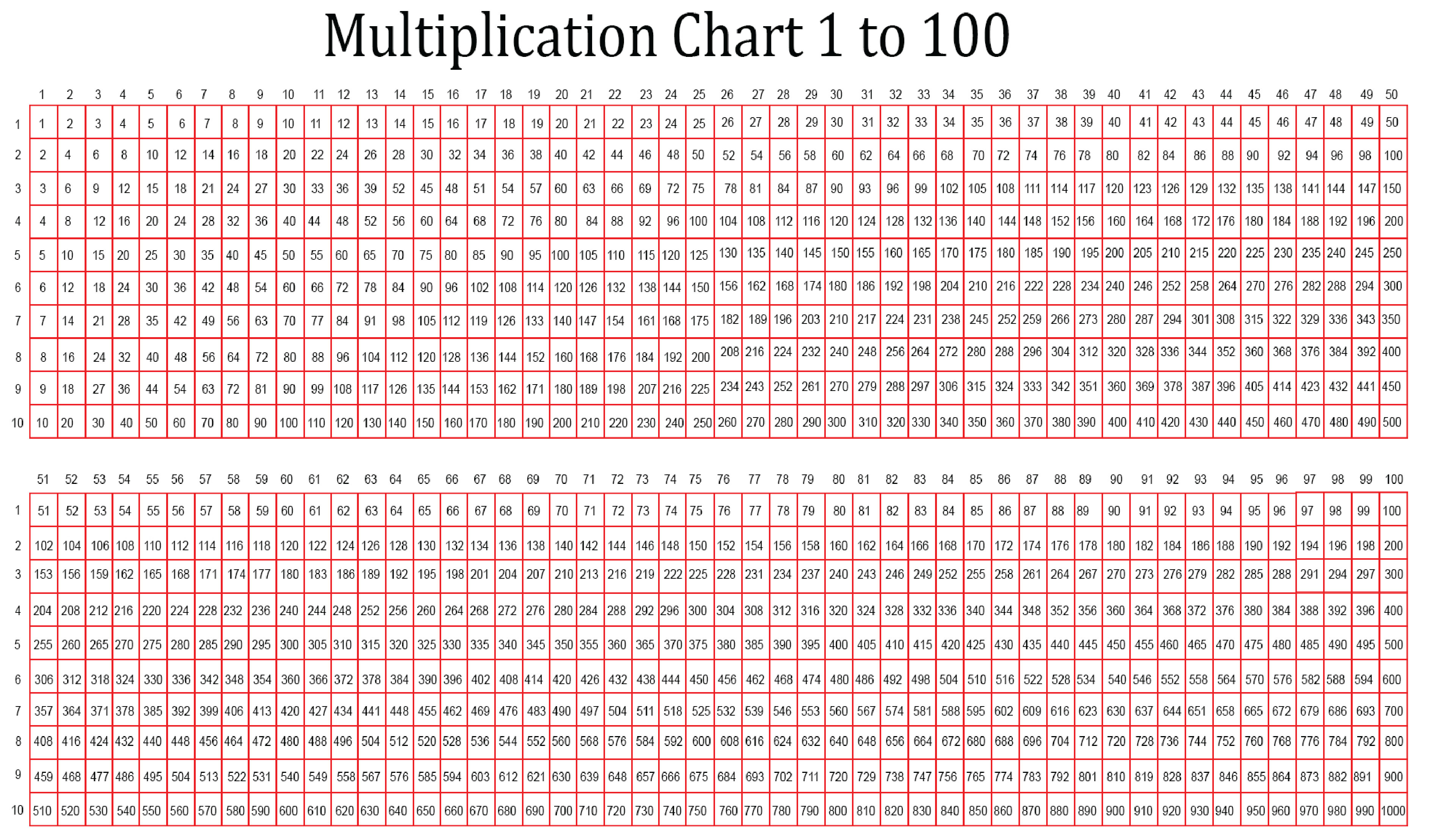We also have more printable chart you may like:
Printable Number Chart 1 -100
50 Chart Printable
Printable Number Chart 1 -120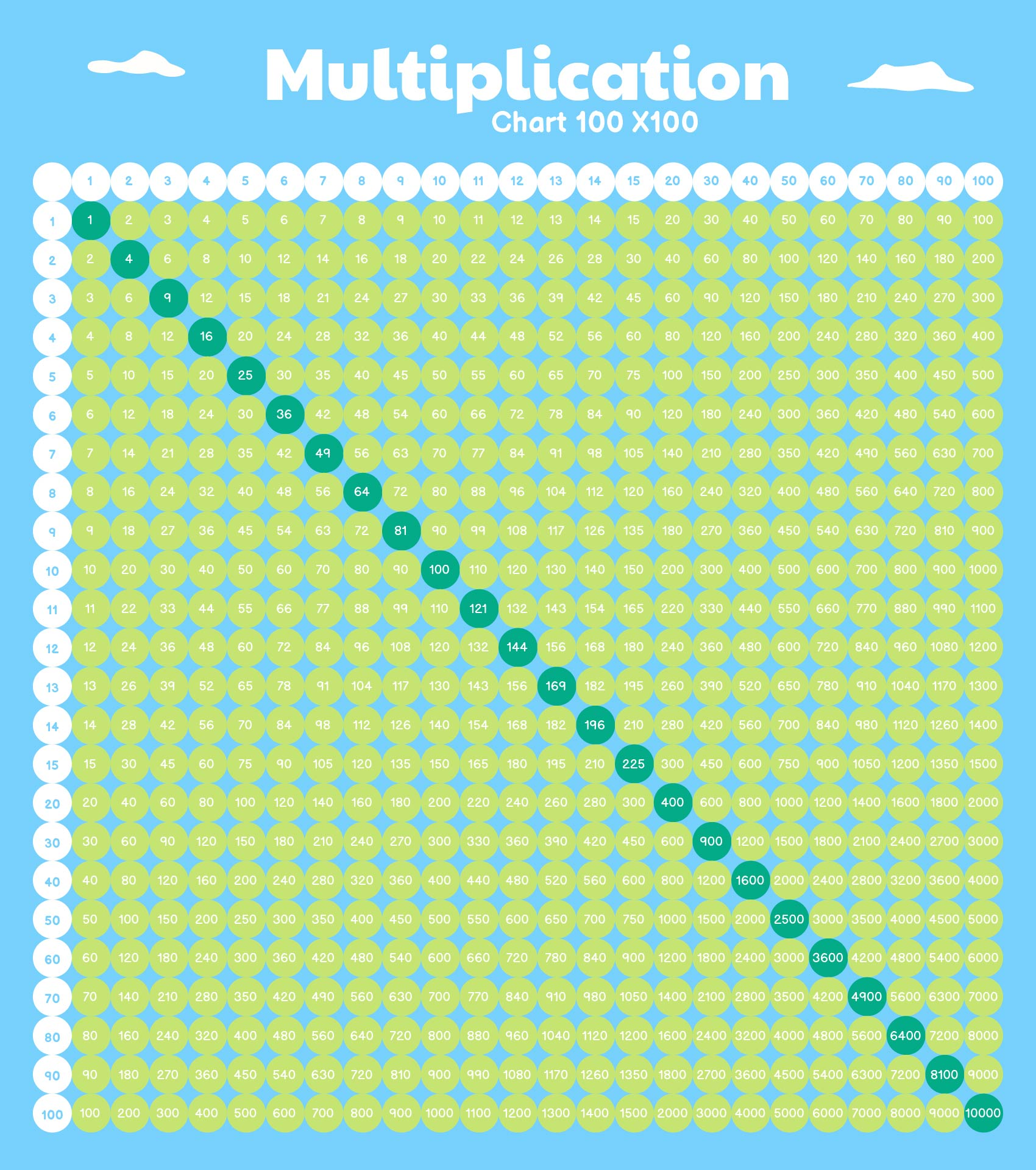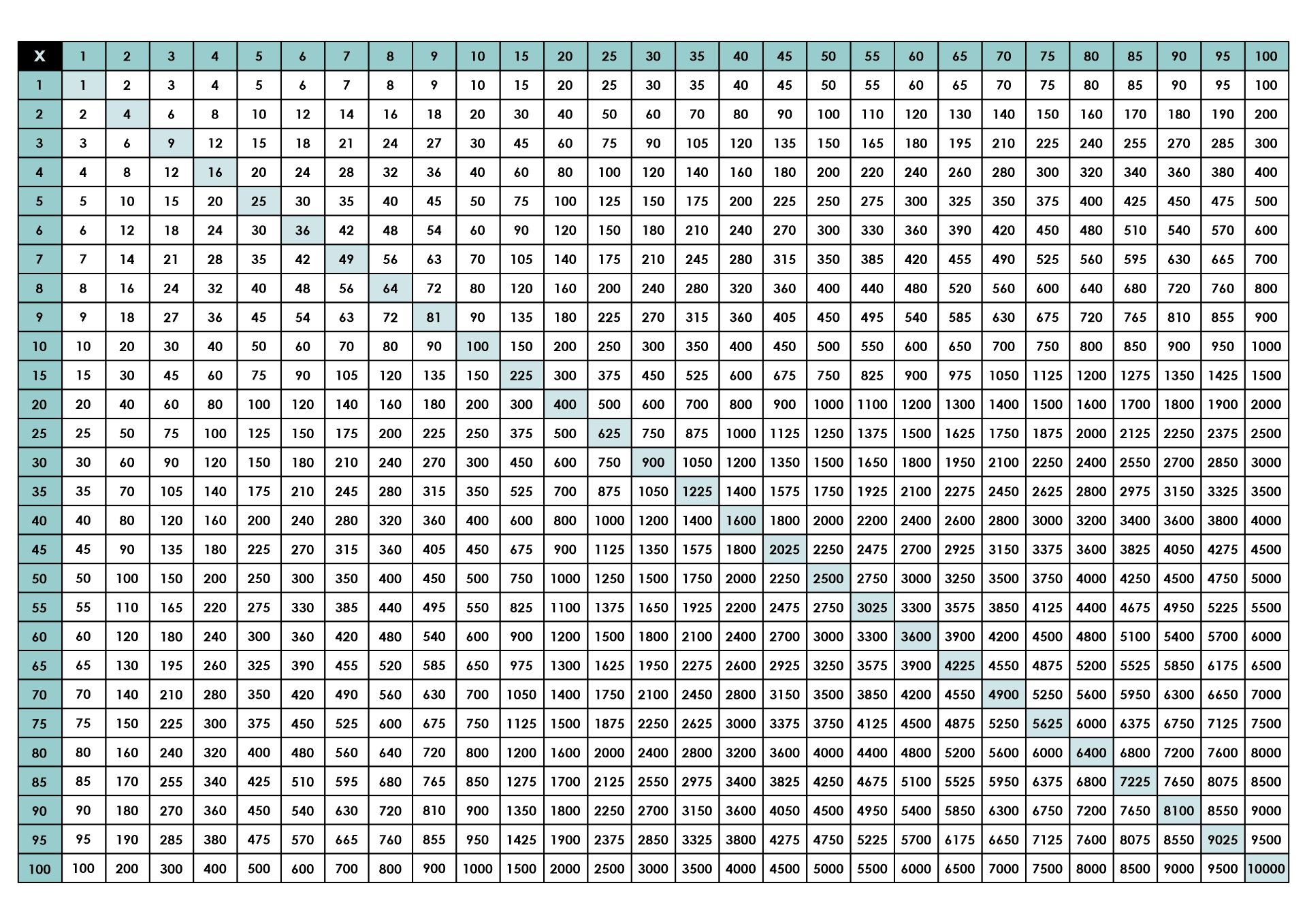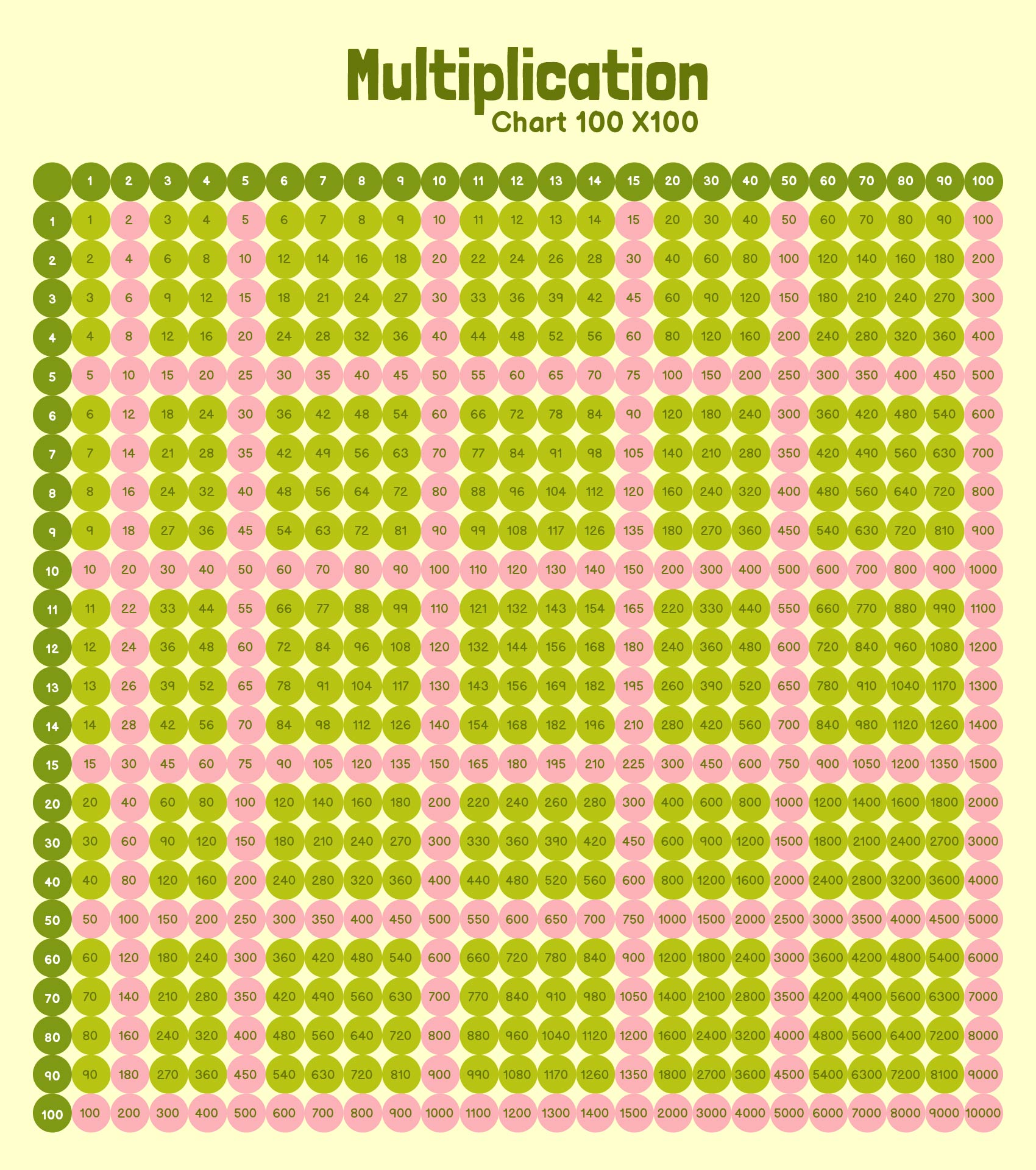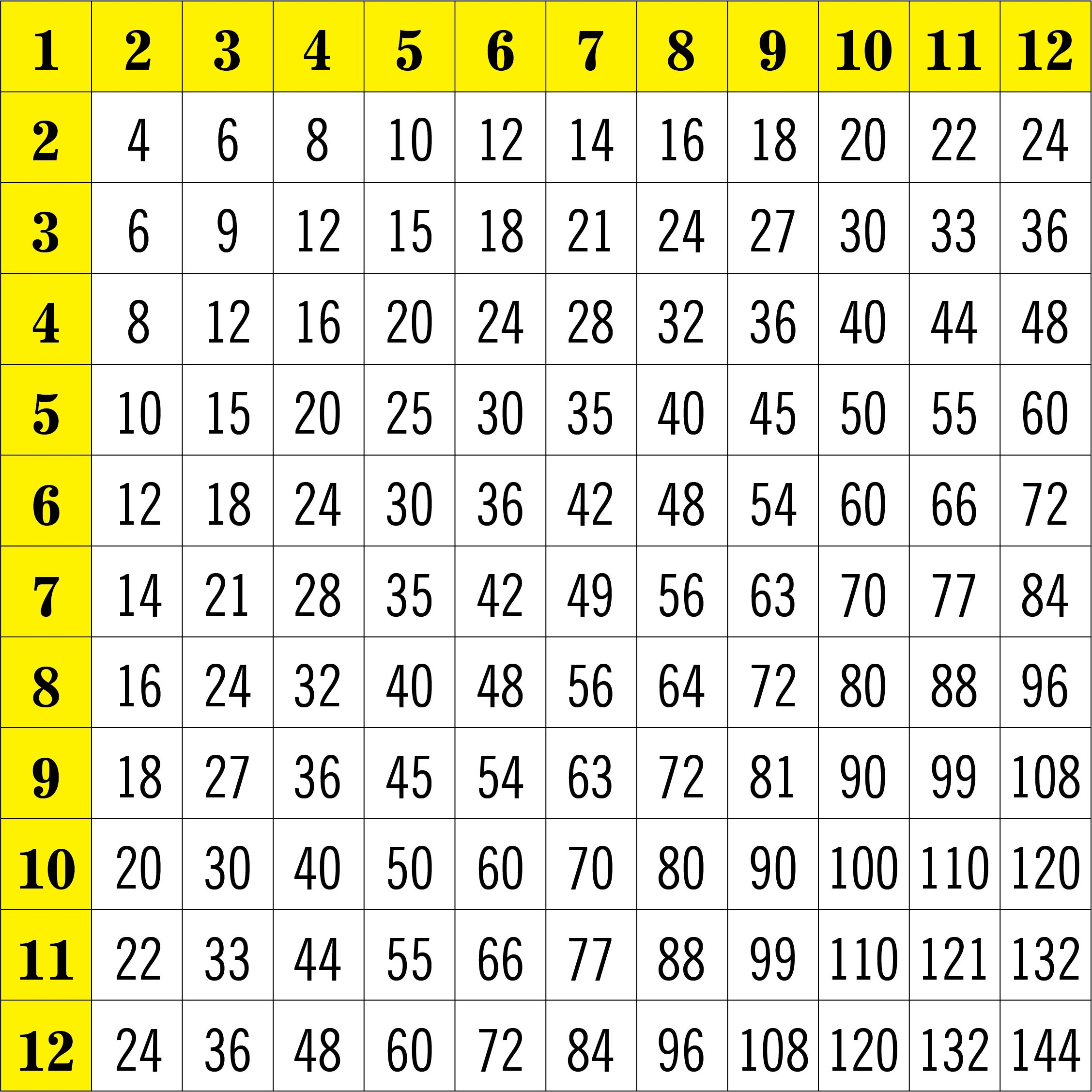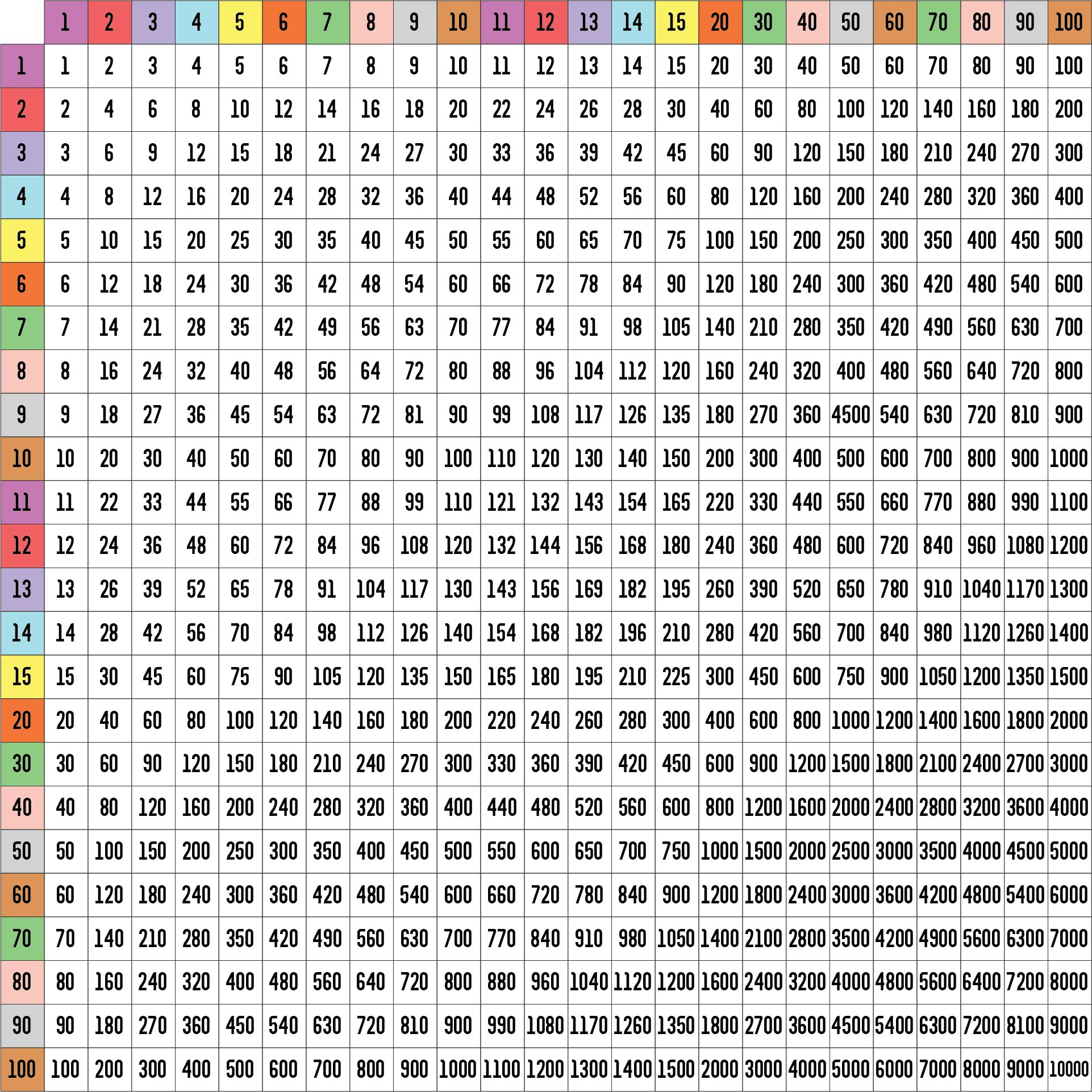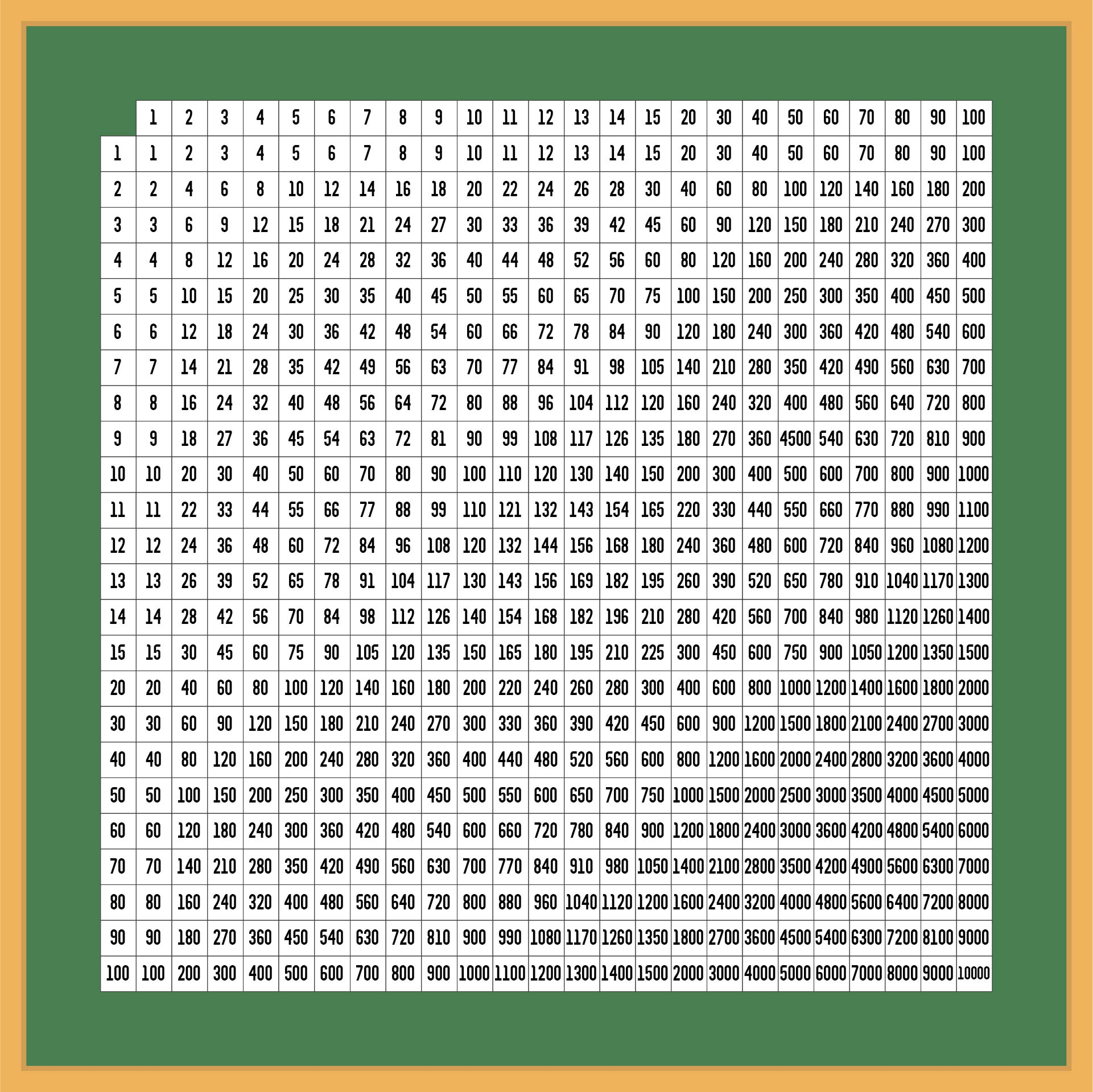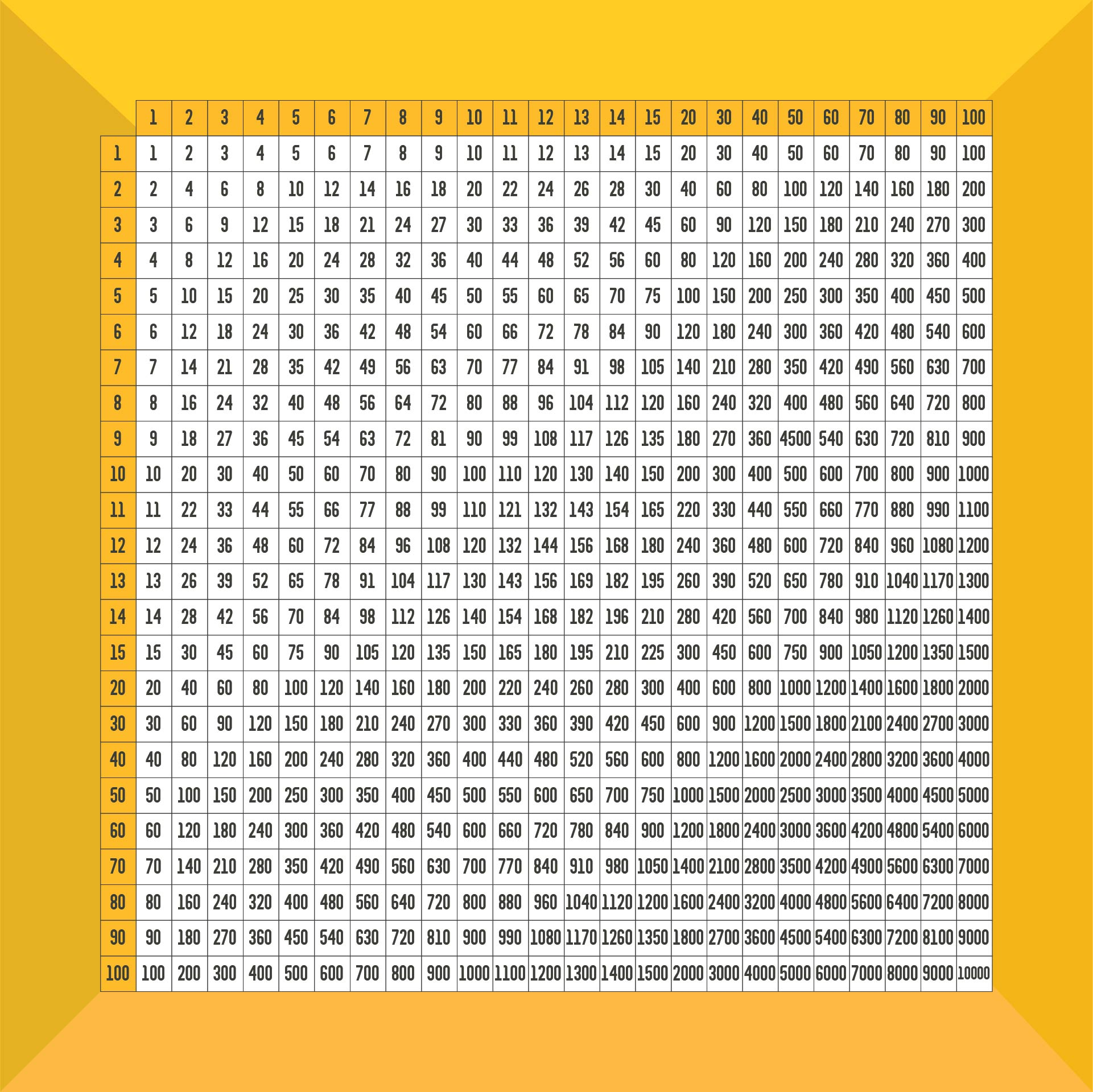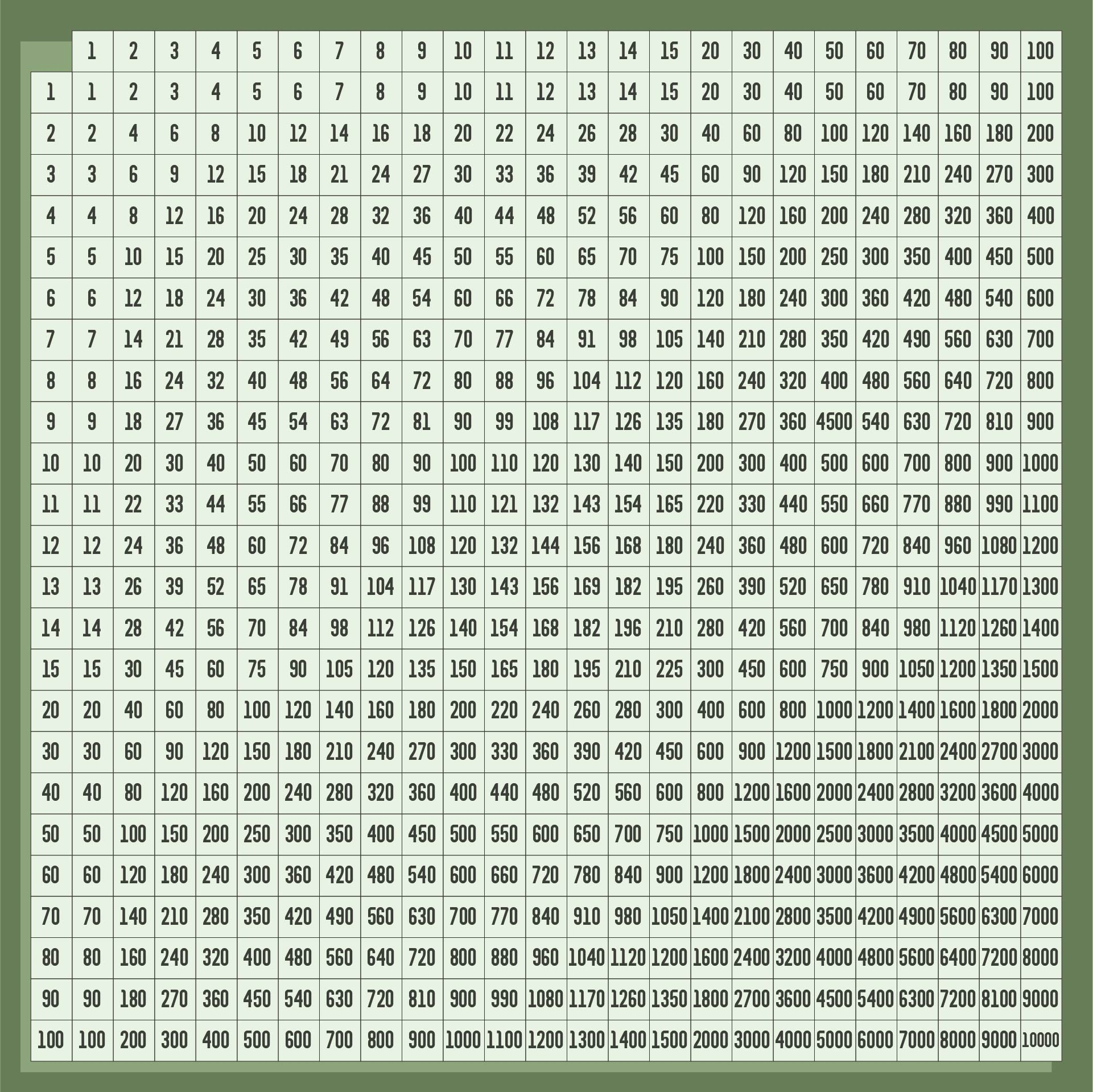## How Does Mathematics Apply in Life?

Our regular activities frequently involve basic mathematical calculations without our even being aware of it. We use mathematical ideas and abilities to grasp time, patterns, and statistics, as well as to calculate cooking measurements, plan travel routes, and budget our money. It supports our ability to solve issues, make wise decisions, and make sense of the world.

Mathematical patterns are closely related to the beauty of nature. Mathematical rules are followed by the hypnotic spirals of seashells, the fractal architectures of ferns, and the symmetry of flower petals. The intricate patterns observed in nature are created by mathematical principles such as Fibonacci sequences, golden ratios, and fractal geometry.

Mathematics is the engine that drives innovation and technology. Data analysis, coding languages, and sophisticated algorithms all have their roots in mathematics. Mathematics offers the basis for discoveries and improvements that form our modern world, from computer graphics to cryptography, from artificial intelligence to medical imaging.

Mathematics has a significant effect on both art and design. Geometric concepts are used by architects to design sturdy and aesthetically pleasing structures. To produce harmonious compositions, artists use mathematical ideas like perspective, symmetry, and proportion. In addition to enhancing aesthetics, mathematics in art allows for exact calculations and rigorous design.

The problem-solving abilities through mathematics go beyond calculating equations. It promotes analytical thinking, critical reasoning, and logical thinking. Mathematical training fosters the capacity to deconstruct difficult issues, spot patterns, and come up with answers. This approach to problem-solving improves our skills in many facets of life, from overcoming obstacles in our daily lives to answering scientific questions.

### What is a multiplication chart?

The multiplication chart as visual aid is useful and could be a resource for learning about and using multiplication. It offers a well-organized breakdown of the results of multiplying numbers, assisting with computations and promoting a deeper comprehension of multiplication ideas.

Multiplication is a critical component of financial planning and computations. A multiplication chart may be a useful tool for doing rapid calculations, whether you're working on interest rates, loan repayments, or investment returns.